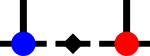SyTen## ◆ pseudo_invert()

 std::tuple< SRDef, Tensor< 2 > > syten::pseudo_invert ( Tensor< 2 > const & a )

Calculates the SVD-based pseudo-inverse of the input matrix.

Given an input a which is decomposed as U·S·Vh, returns a tuple <condition_number,V·1/S·Uh>. The singular value matrix S is inverted using invertDiagonal which applies a broadening

If a is square and has full rank (condition number nonzero) then the returned matrix is close to the exact inverse.

Leg ordering is identical to the input, i.e. the first leg of a should be connected to the second leg of the result or the second leg of a should be connected to the first leg of the result to calculate R·A and A·R respectively.

References diagonal(), invertDiagonal(), norm(), syten::SVD::svd(), and SYTEN_SMALL_THRESHOLD.Here is the call graph for this function:Here is the caller graph for this function: